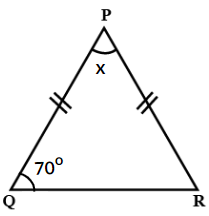Find the value of x in the shown figure.

# Find the value of x in the shown figure.1. A

30o

2. B

40o

3. C

45o

4. D

50o

Fill Out the Form for Expert Academic Guidance!l

+91

Live ClassesBooksTest SeriesSelf Learning

Verify OTP Code (required)

### Solution:

We know that in an isosceles triangle, the angles opposite the equal sides are equal.

Since ΔPQR is an isosceles with PQ = PR.

∴ ∠Q = ∠R = 70o

By the angle sum property, we have

∠P + ∠Q + ∠R = 180o

⇒ x + 70o + 70o = 180o

⇒ x + 140o = 180o

⇒ x = 180o − 140o

⇒ x = 40o

## Related content

 Area of Square Area of Isosceles Triangle Pythagoras Theorem Triangle Formula Perimeter of Triangle Formula Area Formulae Volume of Cone Formula Matrices and Determinants_mathematics Critical Points Solved Examples Type of relations_mathematics+91

Live ClassesBooksTest SeriesSelf Learning

Verify OTP Code (required)